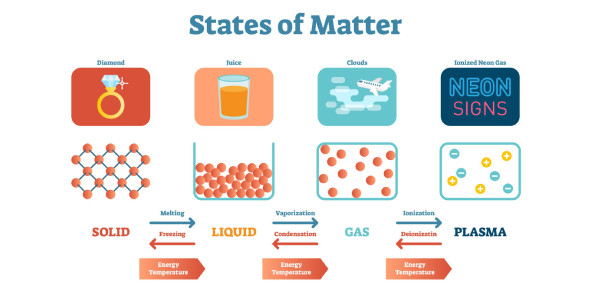# The Best States Of Matter Quiz

10 Questions | Total Attempts: 38871SettingsDo you know there are five known phases, or states, of the matter: solids, liquids, gases, plasma, and Bose-Einstein condensates? The main difference in the structures of each state is in the densities of the particles. Well, what do you know about the topic? Test your knowledge with this quiz.

• 1.
1.  How many states of matter exist?
• A.

1

• B.

2

• C.

3

• D.

4

• E.

5

• 2.
What state of matter are the particles the farthest apart?
• A.

Solid

• B.

Liquid

• C.

Gas

• D.

Plasma

• 3.
What state of matter are the particles the closest together?
• A.

Liquid

• B.

Gas

• C.

Plasma

• D.

Solid

• 4.
What state of matter are the particles in this picture?
• A.

Solid

• B.

Liquid

• C.

Gas

• D.

Plasma

• 5.
What state of matter are the particles in this picture?
• A.

Solid

• B.

Liquid

• C.

Gas

• D.

Plasma

• 6.
What state of matter is rain?
• A.

Solid

• B.

Liquid

• C.

Gas

• D.

Plasma

• 7.
What state of matter has no shape or volume?
• A.

Solid

• B.

Liquid

• C.

Gas

• D.

Plasma

• 8.
What state of matter are the particles in this picture of?
• A.

Solid

• B.

Liquid

• C.

Gas

• D.

Plasma

• 9.
What state of matter is water?
• A.

Solid

• B.

Liquid

• C.

Gas

• D.

All of the above

• 10.
What state of matter are the particles moving in one direction?
• A.

Solid

• B.

Liquid

• C.

Gas

• D.

Plasma

Related TopicsBack to top